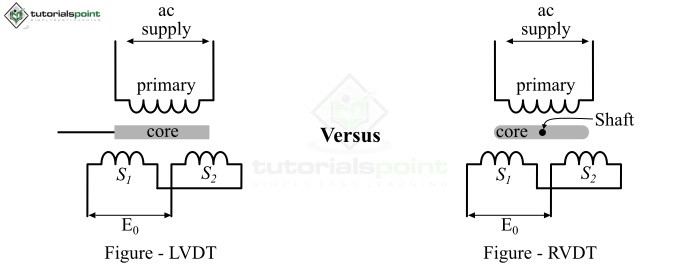# Difference between Linear Variable and Rotary Variable Differential Transformers (LVDT vs RVDT)

LVDT and RVDT are types of passive transducers that convert displacement into an electric signal. Both LVDT and RVDT are types of inductive transducers and their construction is just like a transformer.In this article, we will highlight all the differences between LVDT and RVDT. Let's start with some basics so that it becomes easier to understand the differences between them.

## What is an LVDT?

LVDT stands for Linear Variable Differential Transformer. It is a passive inductive transducer that converts linear displacement into electrical signal for measurement purposes.

A typical LVDT consists of a primary winding, two secondary windings and rectangular shaped core which can move linearly within the former. Therefore, LVDT is a transducer but its basic construction is just like a transformer, and so its name.

An alternating current supply is provided to the primary winding of the LVDT while the two secondary windings are kept at an equal distance on both sides of the primary winding. When the core of the LVDT is moved in different directions linearly, it produces a differential voltage at the output terminals.

Basically, the input AC supply to primary winding of the LVDT produces an alternating magnetic field. This changing magnetic field induces a voltage in the two secondary windings of the LVDT. The movement of the LVDT core causes a variation in the generated voltage in the secondary winding.

Thus, based on the core movement, there can be three different cases of LVDT operation as −

• When the core is present at is normal position, then the voltage induced in the two secondary windings will be equal. Hence, the resultant differential voltage at the output will be zero.

• When the core of LVDT is shifted towards left of the normal position. In this case, the magnetic flux linking to secondary winding 𝑆S1 is greater than that of S2. Consequently, the voltage produced in 𝑆S1 is more than that in the S2. Therefore, the differential voltage at output is given by, $$E_o\:=\:E_{s1}\:-\:E_{s2}$$

• When the core is shifted towards right of from the normal position, in this case the flux linked to the 𝑆S2 is greater than that of S1. Therefore, the differential voltage at the output is given by,

$$E_o\:=\:E_{s2}\:-\:E_{s1}$$

Hence, from these operating cases of LVDT, it is clear that the change in the differential voltage at the output of an LVDT is proportional to the displacement of the core.

## What is an RVDT?

RVDT stands for Rotatory Variable Differential Transformer. The RVDT is also an inductive type passive transducer which converts the angular displacement into an electric signal.

Basically, the construction and working of an RVDT is somewhat similar to an LVDT. However, an RVDT consists of a cam-shaped core that can rotate freely between the windings of the RVDT.

The operation of an RVDT can be understood by considering the following three cases:

• When the core is placed at the null position. The differential voltage at the output will be zero, it is because the voltage induced in the two secondary windings is equal.

• When the core of the RVDT is rotated in the clockwise direction. In this case, the flux linked to the secondary winding S1 is greater than that of S2. Hence, the differential voltage at the output is, $$E_o\:=\:E_{s1}\:-\:E_{s2}$$

• When the core is rotated in the anti-clockwise direction, in this case the flux linked to the S2 is greater than that of S1. Therefore, the differential voltage at the output is given by, $$E_o\:=\:E_{s2}\:-\:E_{s1}$$

Hence, it is clear that the differential voltage at the output of an RVDT is directly proportional to angular displacement of the core.

## Difference between LVDT and RVDT

Both LVDT and RVDT are types of passive transducers used for measuring displacement. However, there are several differences between LVDT and RVDT that are given in the following table

Basis of Difference LVDT RVDT
Full form LVDT stands for Linear Variable Differential Transformer. RVDT stands for Rotatory Variable Differential Transformer.
Definition LVDT is a type of passive transducer which converts the linear displacement into electrical signal. RVDT is a type of passive transducer which converts the angular displacement into electrical signals.
Shape of transformer core LVDT has a rectangular shaped core RVDT has a cam shaped core.
Movement of core In LVDT, the movement of core is linear. In RVDT, the movement of core is circular.
Measurement factor LVDT is used to measure linear displacement. RVDT is used to measure angular displacement.
Measurement range LVDT can measure the linear displacement in the range of ± 100 μm to ± 25 cm. RVDT can measure the angular displacement in the range of + 40° to – 40°.
Input voltage The RMS value of the input voltage of a typical LVDT is ranging from 1 V to 24 V. The RMS value of input voltage of an RVDT is up to 3 V.
Frequency range The operating frequency range of an LVDT is 50 Hz to 20 kHz. The operating frequency range of an RVDT is 400 Hz to 20 kHz.
Sensitivity The sensitivity of an LVDT is comparatively high, and it is approximately equal to 2.4 mV/Volt/unit of movement. The sensitivity of an RVDT is less and its range is from 2 mV to 3 mV per volt per degree of rotation.
Applications LVDT is used in different measuring systems such as weight measurement, pressure measurement, and displacement measurement. It is also used to inspect the products. RVDT is used in several controlling systems such as radar and antenna systems, fire controlling systems and in many other precision controlling applications.

## Conclusion

From the above discussion, we may conclude that LVDT and RVDT are types of passive transducers used for the measurement of linear and angular displacements, respectively.